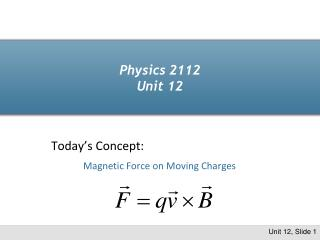DownloadDownload PresentationPhysics 2112 Unit 12

# Physics 2112 Unit 12

Download Presentation## Physics 2112 Unit 12

- - - - - - - - - - - - - - - - - - - - - - - - - - - E N D - - - - - - - - - - - - - - - - - - - - - - - - - - -
##### Presentation Transcript

1. Physics 2112Unit 12 Today’s Concept: Magnetic Force on Moving Charges

2. Magnetic Force • Electric Field • Any particle can cause E field • Any particle affected by E field • Magnetic Field • Only moving particle can cause B field • Only moving particle affected by B field Unit 12, Slide 2

3. Units Units: Telsa(N/(Cm/sec)) Extremely large field so sometimes use Gauss = (10-4T) • Earth ~ 0.6 Gauss • Frig Magnet ~ 0.01T (100 Gauss) • MRI 1.5 ->5.0 Tesla (15,000 – 30,000 Gauss) • LHC 8.4 Tesla • World’s Record 45 Tesla (National High Magnetic Field Laboratory at Florida State) Unit 12, Slide 3

4. Cross Product Review • Cross Product different from Dot Product is a scalar; is a vector proportional to the component of Bparallel to A proportional to the component of Bperpendicular to A • Definition of • Magnitude: |A||B|sinq Direction: perpendicular to plane defined by AandBwith sense given by right-hand-rule Unit 12, Slide 4

5. Remembering Directions: The Right Hand Rule y x F B qv z Unit 12, Slide 5

6. Example 12.1 (Acceleration of proton) The earth’s magnetic field is pointed roughly 70o from the horizontal in Chicago. What would the acceleration of a proton be if it were moving at 1% of the speed of light in the horizontal direction? Unit 12, Slide 6

7. CheckPoint 2 Three points are arranged in a uniform magnetic field. The B field points into the screen. A positively charged particle is located at point A and is stationary. The direction of the magnetic force on the particle is: right left into the screen out of the screen zero Unit 12, Slide 7

8. CheckPoint3 Three points are arranged in a uniform magnetic field. The B field points into the screen. Now the positive charge moves from point A toward B. The direction of the magnetic force on the particle is: right left into the screen out of the screen zero Unit 12, Slide 8

9. Motion of Charge q in Uniform B Field v x xxxxxx x xxxxxx x xxxxxx x xxxxxx x xxxxxx x xxxxxx F F v v F F v • Force is perpendicular to v • Speed does not change • Uniform Circular Motion R • Solve for R: Uniform B into page q q q q Demo Unit 12, Slide 9

10. Example 12.2 (Analysis Magnets) A proton enters the magnetic field of an analysis magnet at Fermilab and it deflected by an angle of Q = 10o. The field has a strength of 0.2T and a length of 2meters. What is the speed of the incoming proton? Q X XXXXXX X XXXXXX X XXXXXX X XXXXXX X XXXXXX X XXXXXX X XXXXXX Q R sinQ Unit 12, Slide 10

11. Follow up Photograph of track in bubble chamber (pre 1984) Electronic version of same thing (top quark event at CDF) The analysis magnet Question: In the previous example how did you know it was a proton? Unit 12, Slide 11